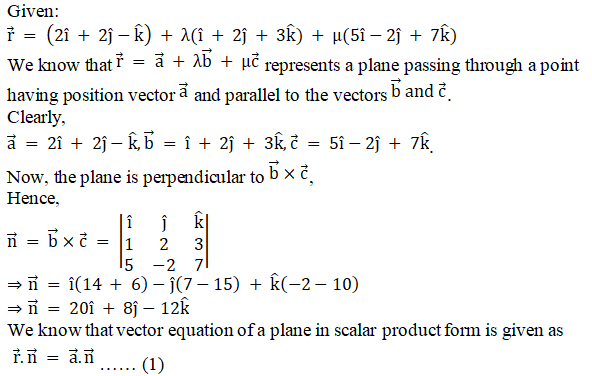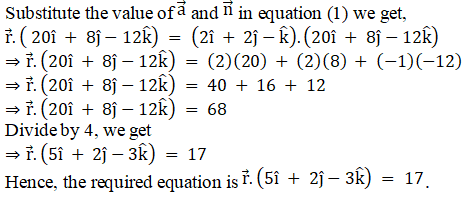# RD Sharma Solutions Class 12 The Plane Exercise 29.7

RD Sharma Solutions for Class 12 Maths Chapter 29 The Plane Exercise 29.7, is provided here for students to prepare and score good marks in the exam. The concepts are explained in detail and solutions are prepared by our expert faculty team in the best possible ways students can understand. Students can refer and easily download the pdf of RD Sharma Class 12 Solutions for free, from the links given below.

## Download PDF of RD Sharma Solutions For Class 12 Maths Chapter 29 Exercise 7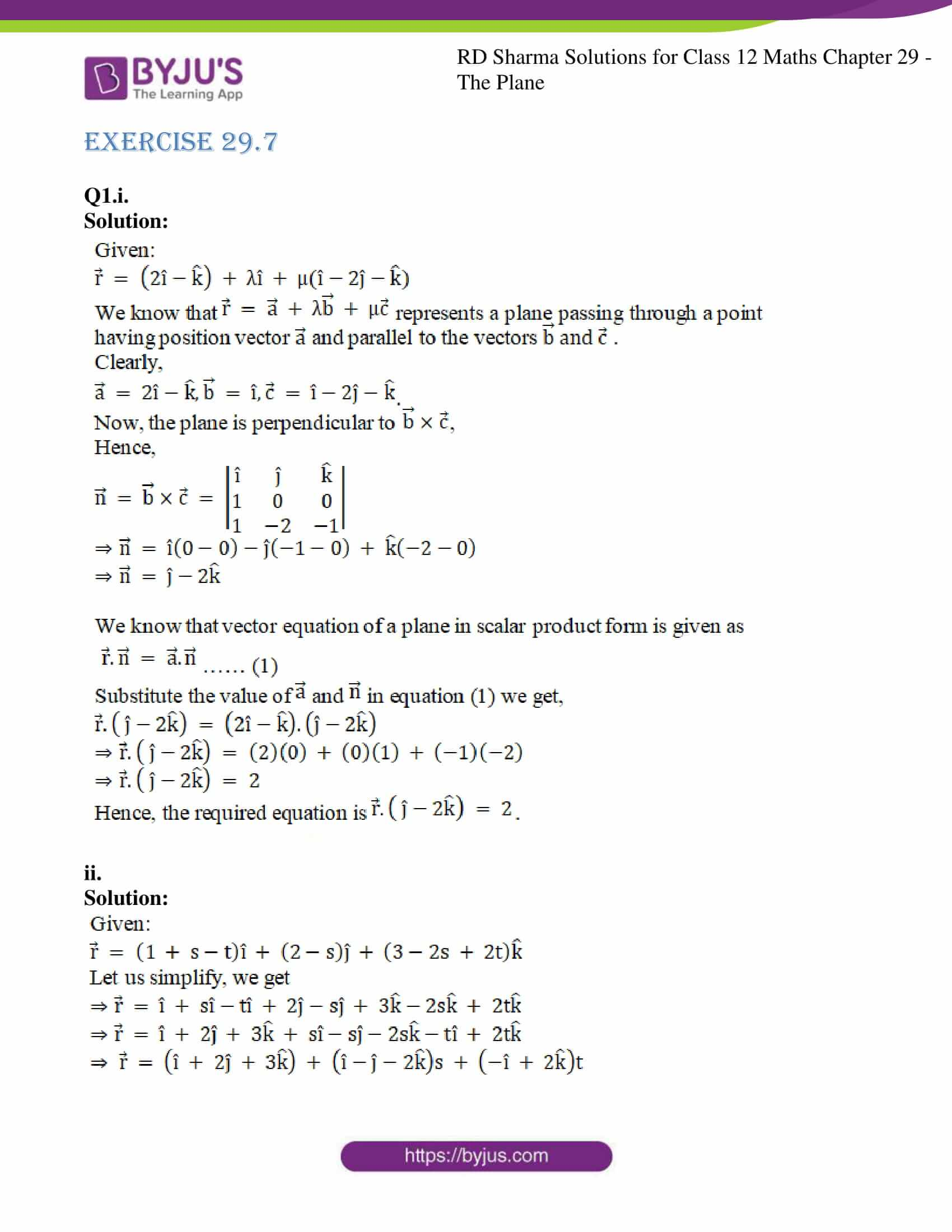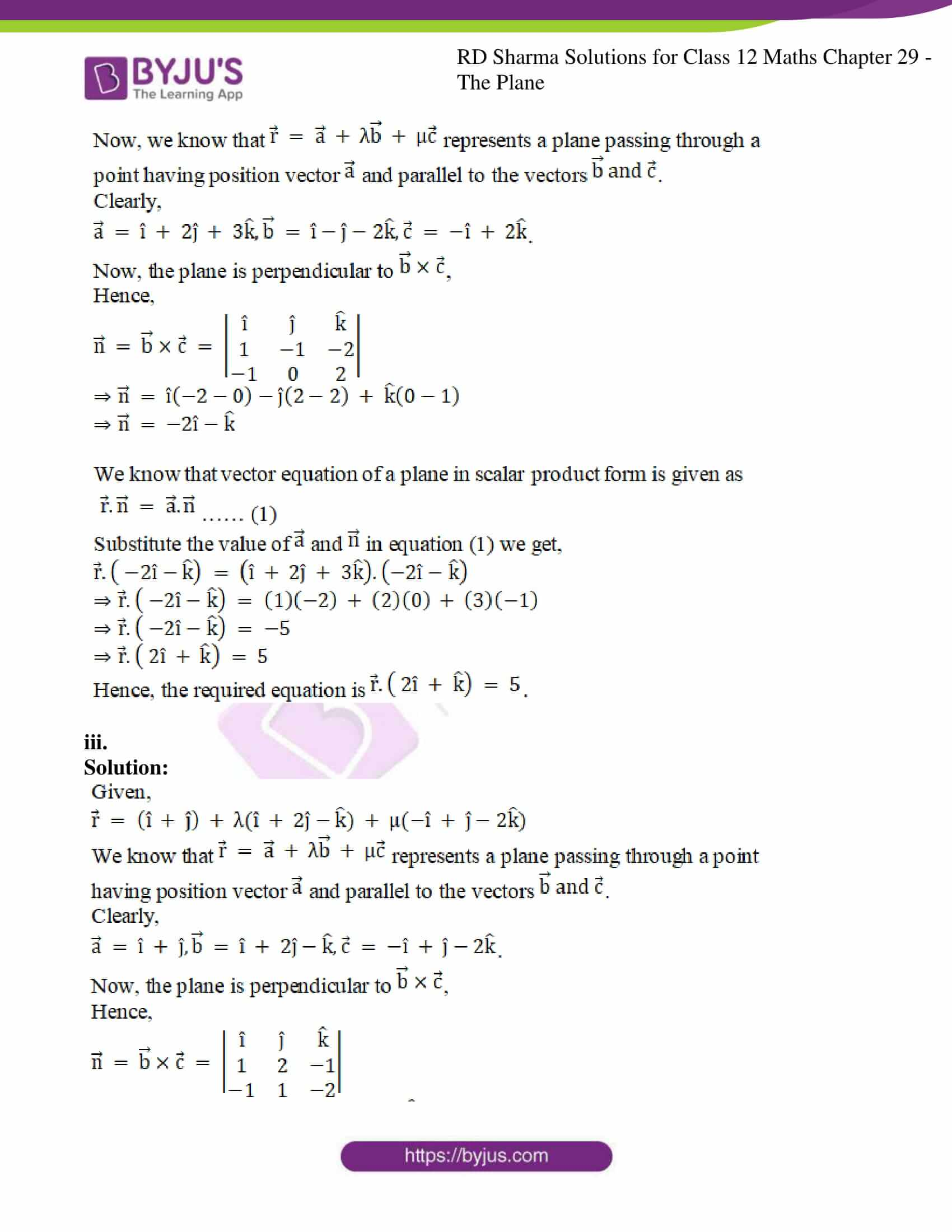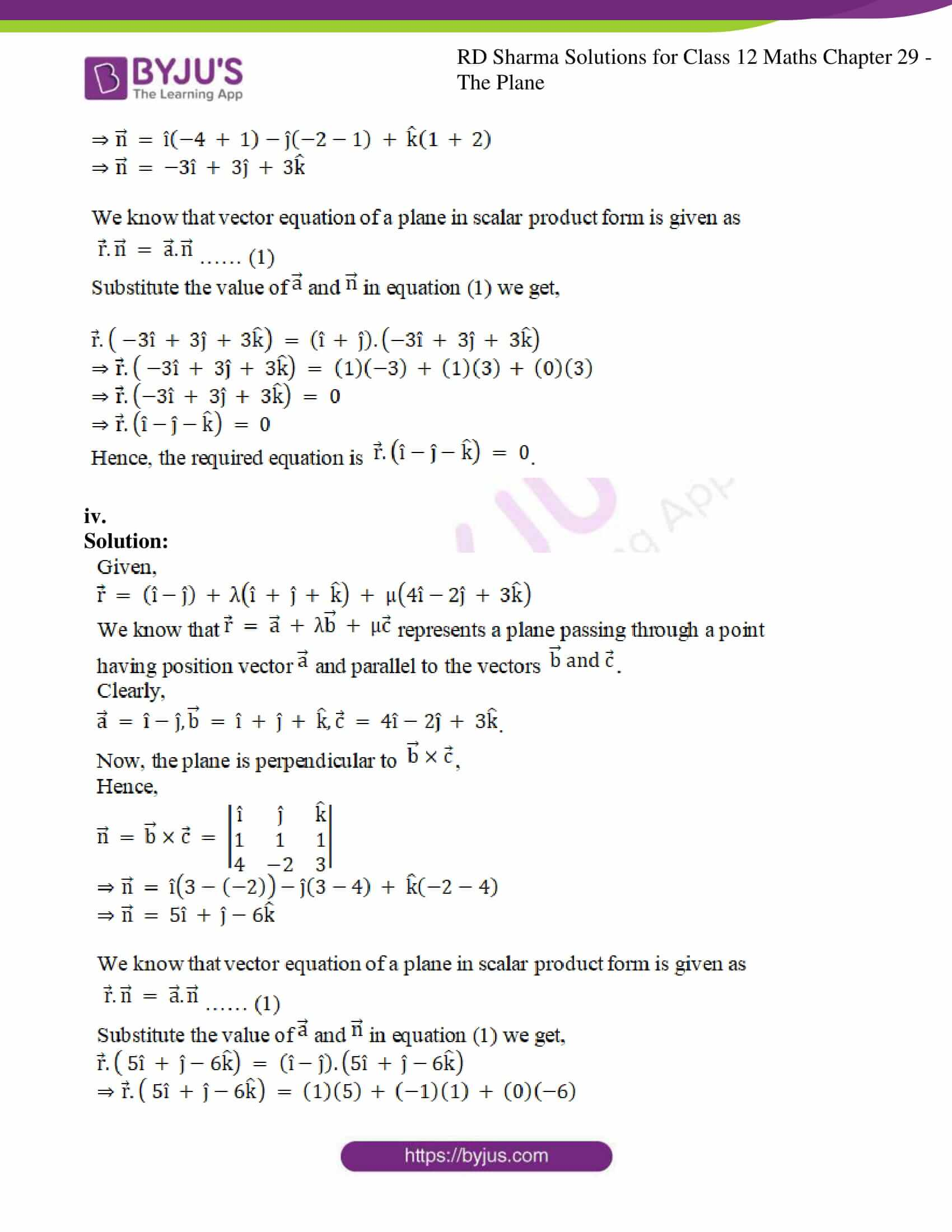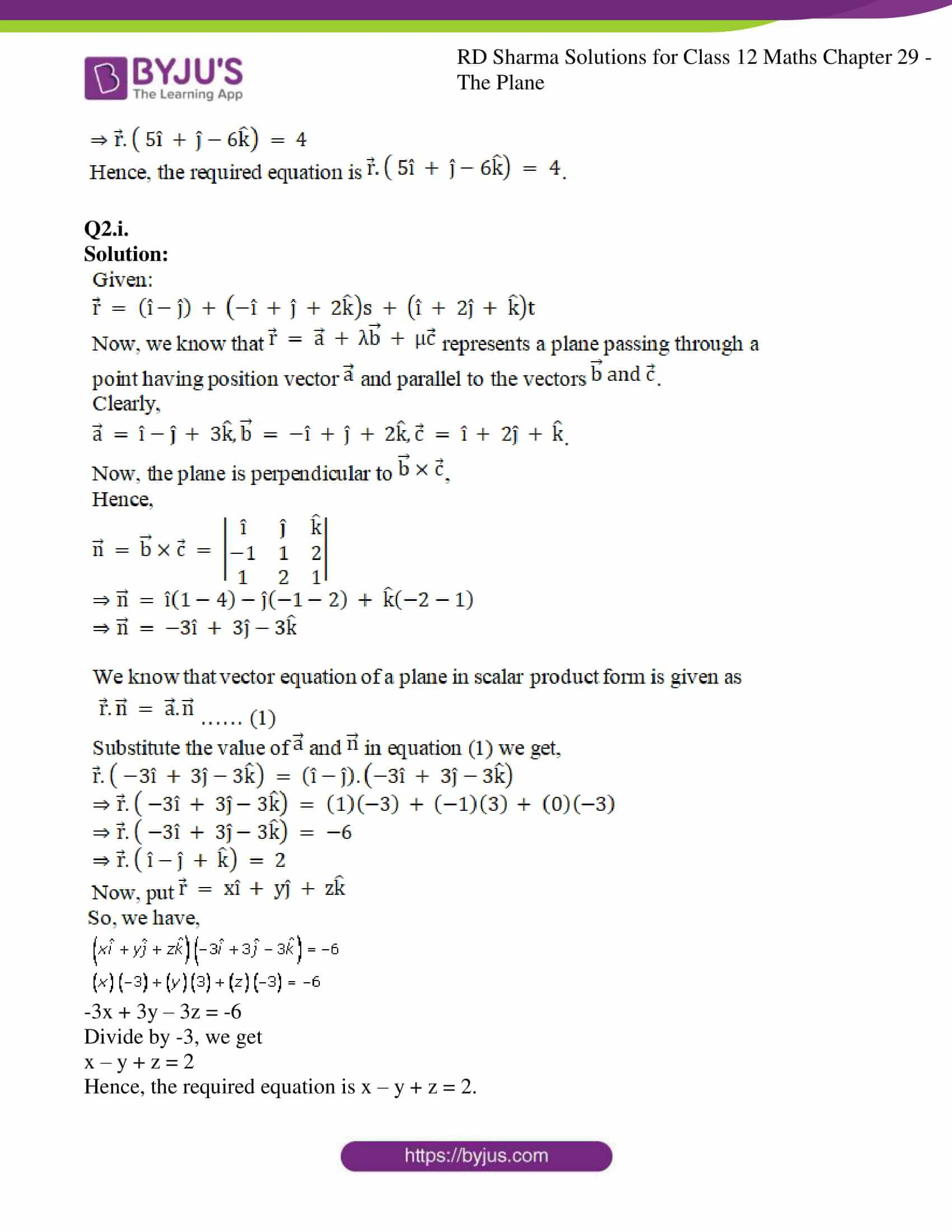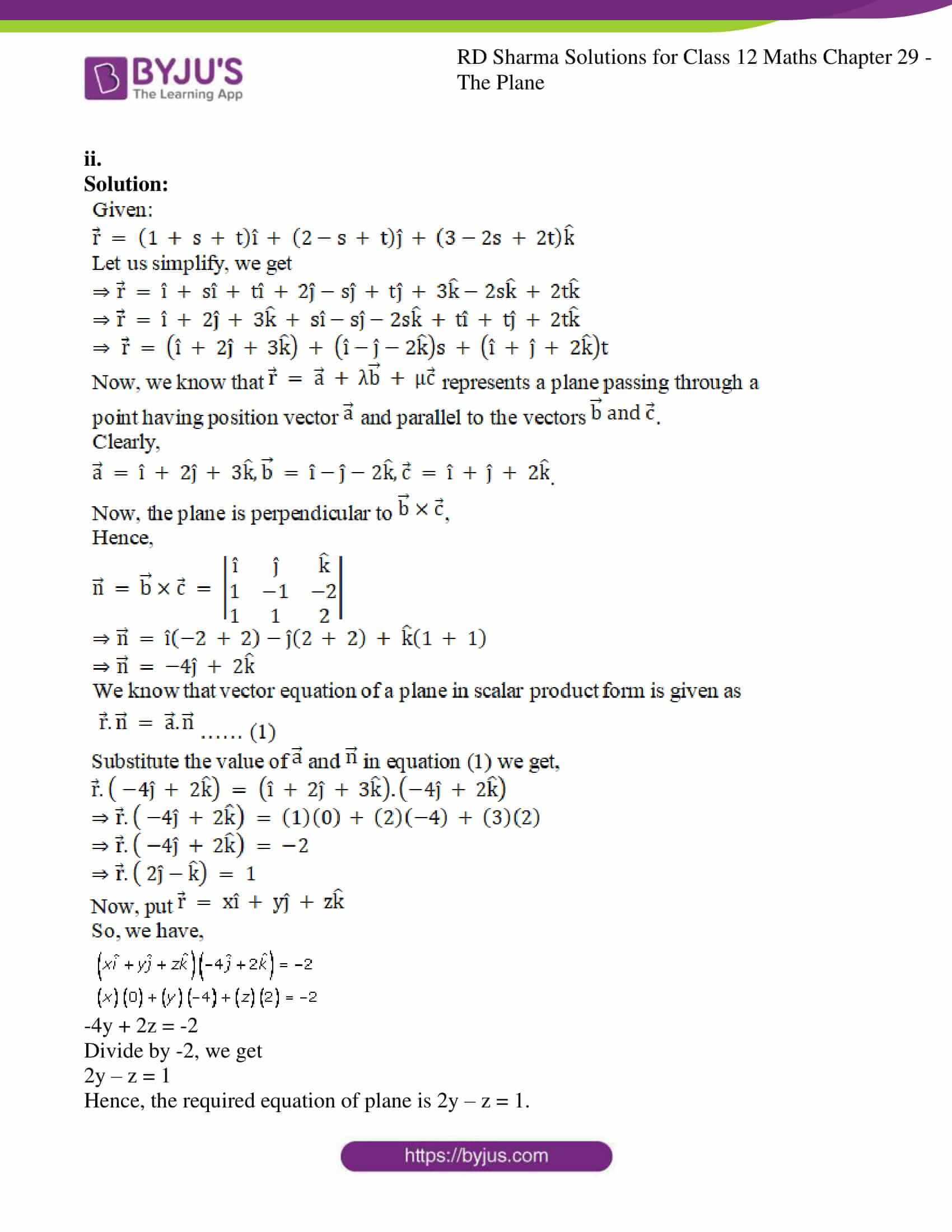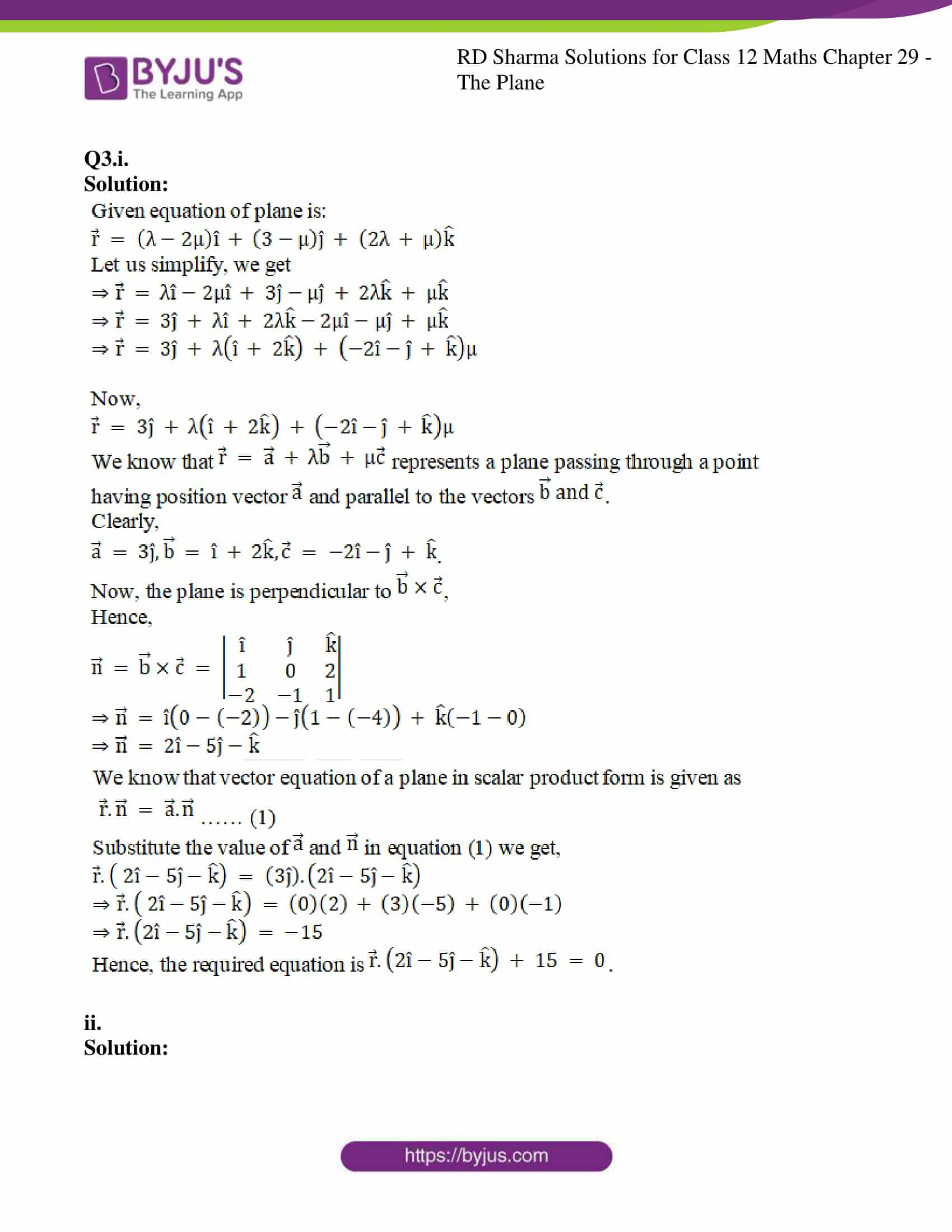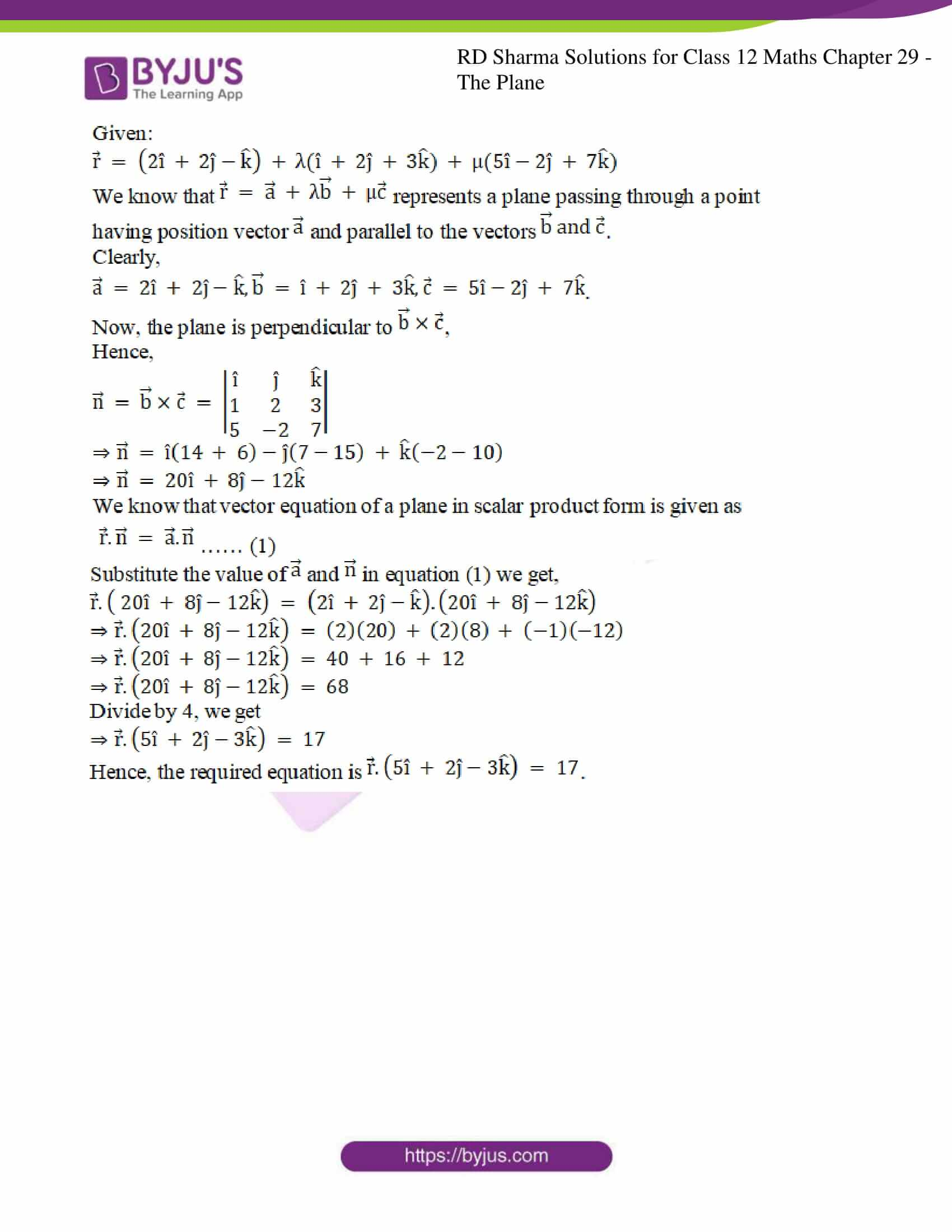### Access RD Sharma Solutions For Class 12 Maths Chapter 29 Exercise 7

EXERCISE 29.7

Q1.i.

Solution: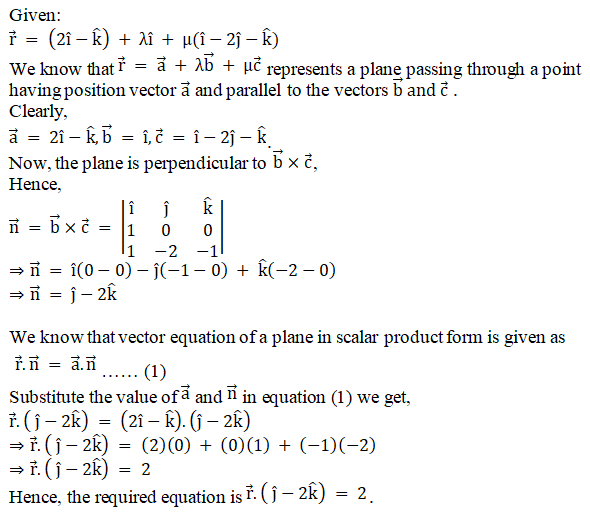ii.

Solution: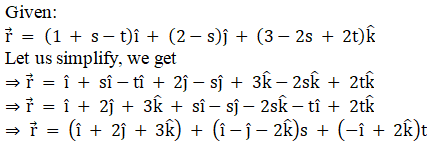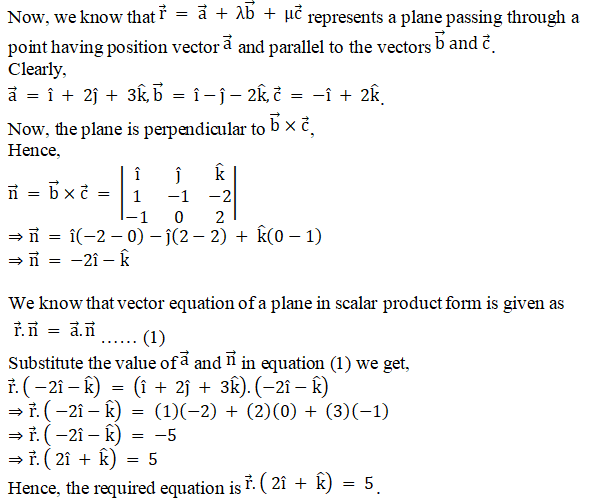iii.

Solution: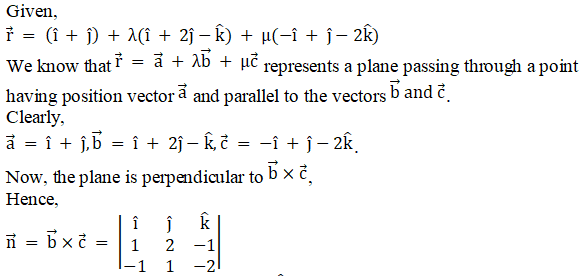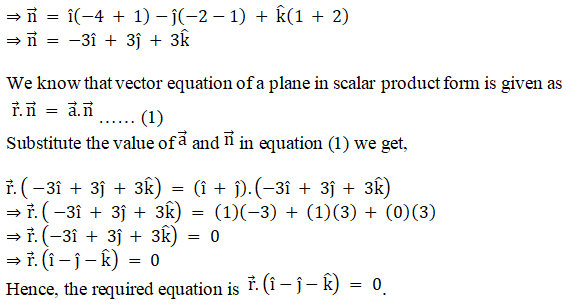iv.

Solution: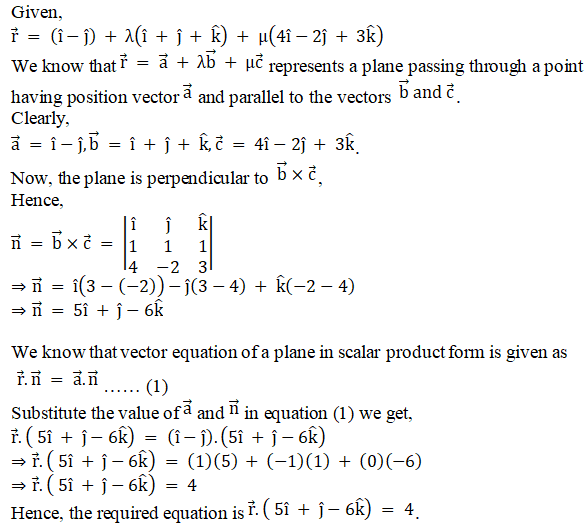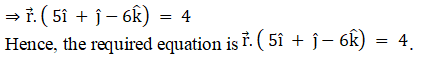Q2.i.

Solution: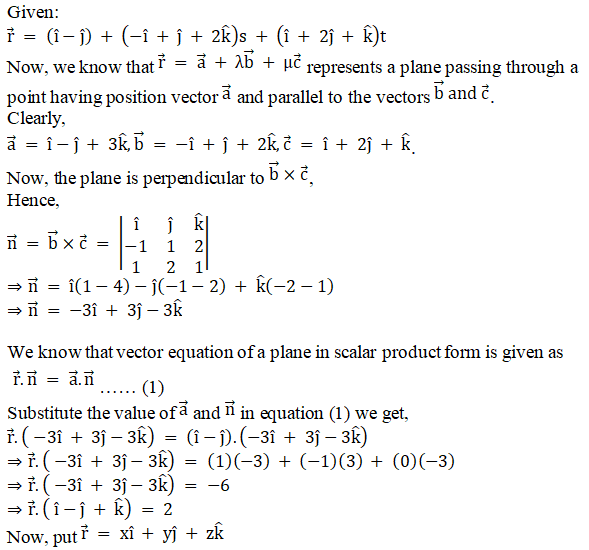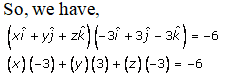-3x + 3y – 3z = -6

Divide by -3, we get

x – y + z = 2

Hence, the required equation is x – y + z = 2.

ii.

Solution: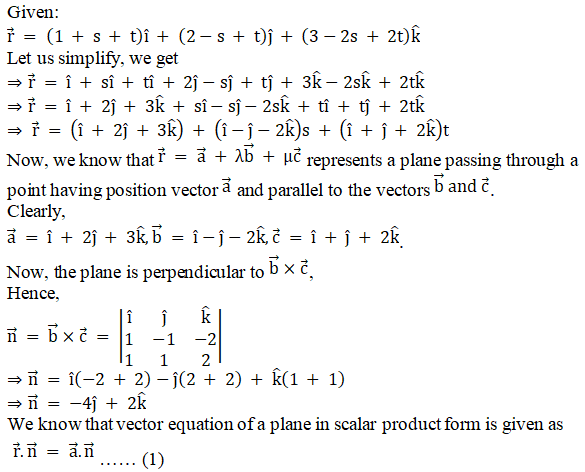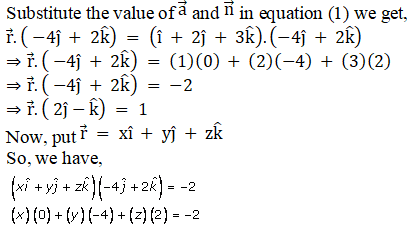-4y + 2z = -2

Divide by -2, we get

2y – z = 1

Hence, the required equation of plane is 2y – z = 1.

Q3.i.

Solution: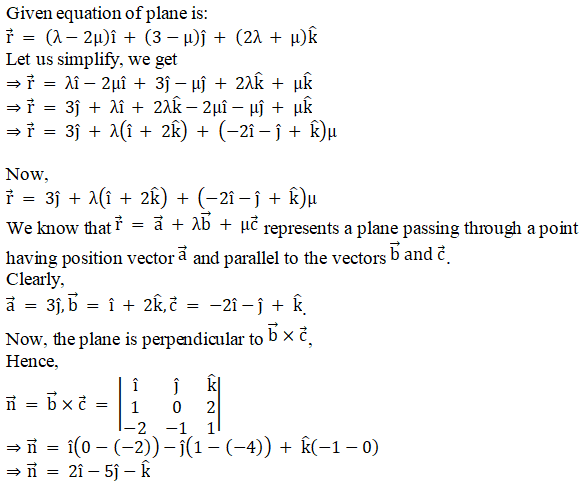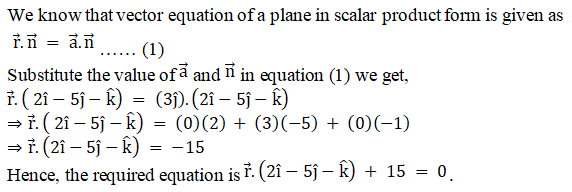ii.

Solution: